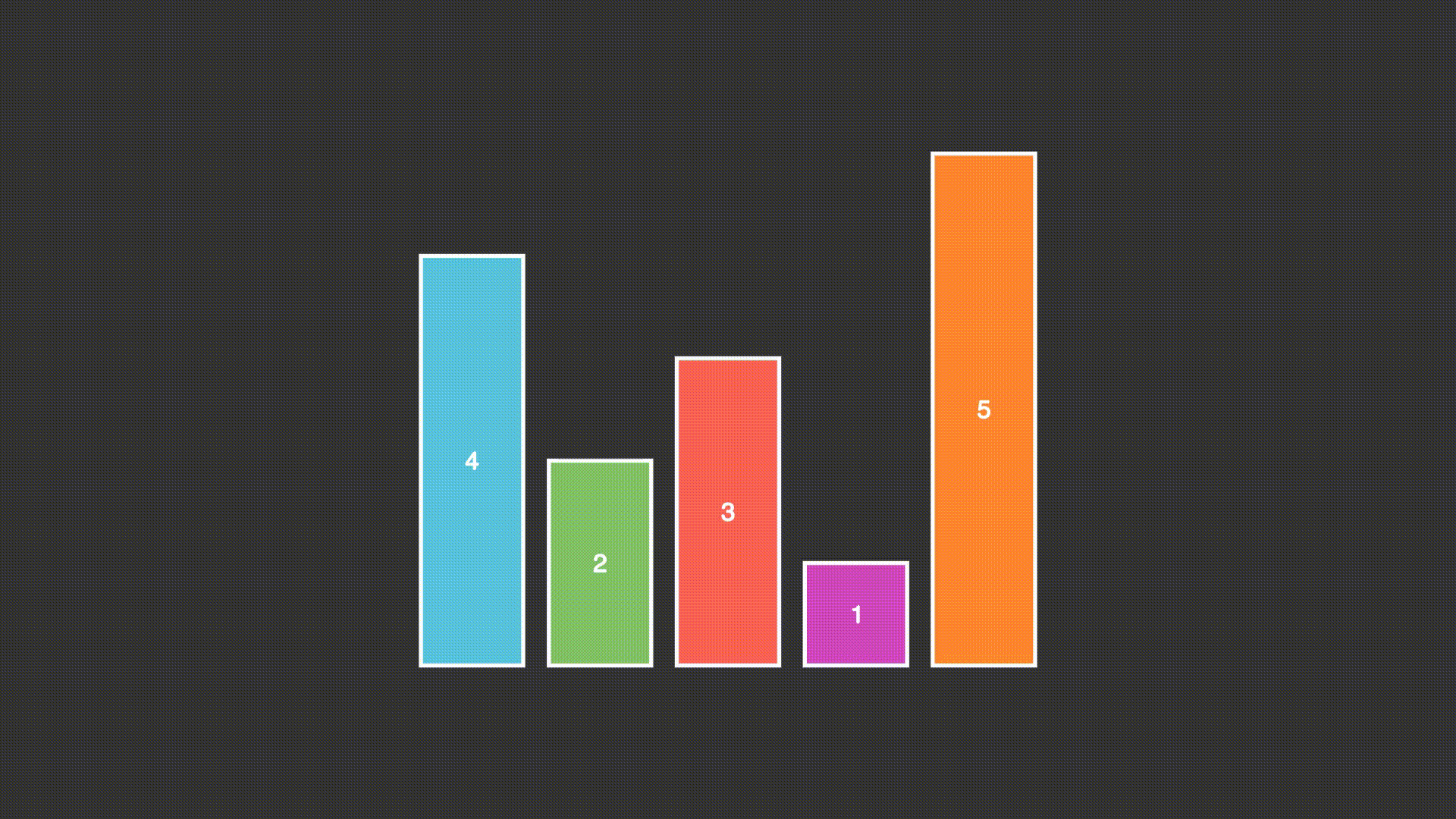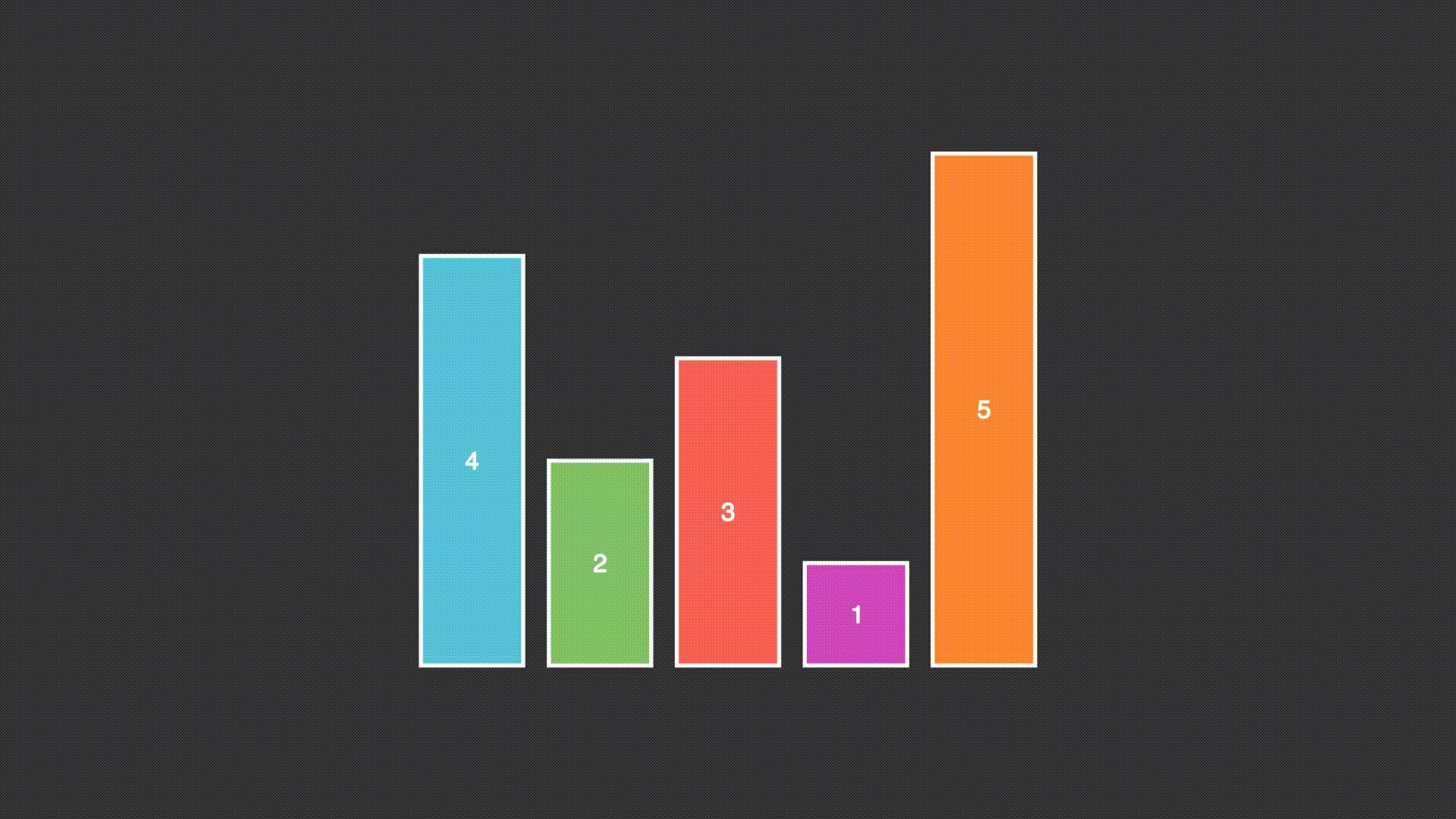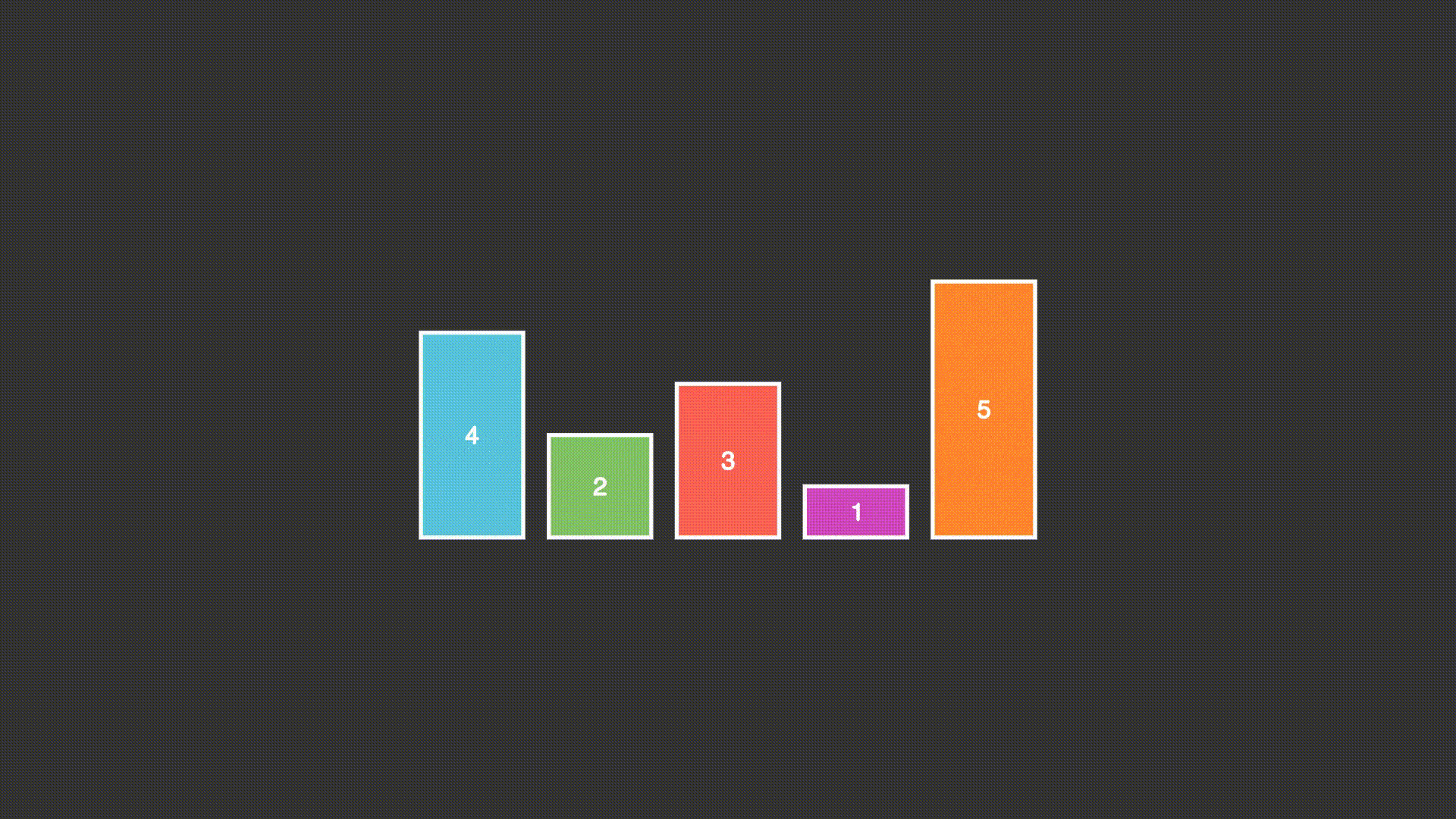# 用 manim 写一个排序算法动画

## 什么是 manim

Manim 是一个用于精确编程动画的引擎，专为创建解释性数学视频而设计。

## 冒泡排序介绍

### 算法步骤

1. 比较相邻的元素。如果第一个比第二个大，就交换他们两个。

2. 对每一对相邻元素作同样的工作，从开始第一对到结尾的最后一对。这步做完后，最后的元素会是最大的数。

3. 针对所有的元素重复以上的步骤，除了最后一个。

4. 持续每次对越来越少的元素重复上面的步骤，直到没有任何一对数字需要比较。

## 初始化元素

• main.py
``````from manimlib import *

class Test(Scene):
def construct(self):
COLOR = [BLUE, GREEN, RED, PINK, ORANGE, MAROON_B, TEAL, PURPLE_B, GREY_BROWN]
arr = [4,2,3,1,5]
g = VGroup()
for i in range(len(arr)):
r1=Rectangle(width=1,height=arr[i],fill_color=COLOR[i%len(COLOR)],fill_opacity=1)
t1=Text(str(arr[i])).scale(0.5)
rec = VGroup(r1,t1)

g.arrange(RIGHT,aligned_edge=DOWN)
self.wait()
``````

``````manimgl main.py BubbleSort
``````
``````ManimGL v1.6.1
[11:27:18] INFO     Using the default configuration file, which you can modify in `/Users/zheng/anaconda3/envs/manim/lib/python3.10/site-packages/manimlib/default_config.yml`                                                                                         config.py:265
INFO     If you want to create a local configuration file, you can create a file named `custom_config.yml`, or run `manimgl --config`                                                                                                                       config.py:266
[11:27:20] INFO     Tips: You are now in the interactive mode. Now you can use the keyboard and the mouse to interact with the scene. Just press `q` if you want to quit.
``````

## 代码说明

`Rectangle` 类定义了矩形的创建，更多图形可以查看 https://docs.manim.org.cn/documentation/mobject/geometry.html#tipablevmobject

## 元素交换动画

``````self.play(CyclicReplace(g, g))
self.wait()
````````````g.generate_target()
g.target.next_to(g,ORIGIN,aligned_edge=DOWN)

g.generate_target()
g.target.next_to(g,ORIGIN,aligned_edge=DOWN)
``````

`generate_target()` 表示生成元素的 target, `next_to()` 表示将元素移动到指定的位置。

``````self.play(MoveToTarget(g),MoveToTarget(g))
``````## 实现代码

``````    self.g[j],self.g[j+1] = self.g[j+1],self.g[j]
TypeError: 'VGroup' object does not support item assignment
``````

``````from manimlib import *

class BubbleSort(Scene):
def construct(self):
self.COLOR = [BLUE, GREEN, RED, PINK, ORANGE, MAROON_B, TEAL, PURPLE_B, GREY_BROWN]
self.bubbleSort([4,2,3,1,5])

def init_vmobj(self,arr):
'''
初始化场景元素
'''
self.vmArr = []
g = VGroup()
for i in range(len(arr)):
r1=Rectangle(width=1,height=arr[i]/2,fill_color=self.COLOR[i%len(self.COLOR)],fill_opacity=1)
t1=Text(str(arr[i])).scale(0.5)
rec = VGroup(r1,t1)
self.vmArr.append(rec)
g.arrange(RIGHT,aligned_edge=DOWN)

self.wait()
def bubbleSort(self,arr):
'''
冒泡排序
'''
self.init_vmobj(arr)
for i in range(1, len(arr)):
for j in range(0, len(arr)-i):
self.play(Indicate(self.vmArr[j]))
self.play(Indicate(self.vmArr[j+1],color=RED))
if arr[j] > arr[j+1]:
arr[j], arr[j + 1] = arr[j + 1], arr[j]
self.cyc_move(self.vmArr[j],self.vmArr[j+1])
self.vmArr[j],self.vmArr[j+1] = self.vmArr[j+1],self.vmArr[j]
return arr
def cyc_move(self,vm1,vm2):
'''
交换两个元素位置
'''
vm1.generate_target()
vm1.target.next_to(vm2,ORIGIN,aligned_edge=DOWN)

vm2.generate_target()
vm2.target.next_to(vm1,ORIGIN,aligned_edge=DOWN)

self.play(MoveToTarget(vm1),MoveToTarget(vm2))
self.wait()
``````